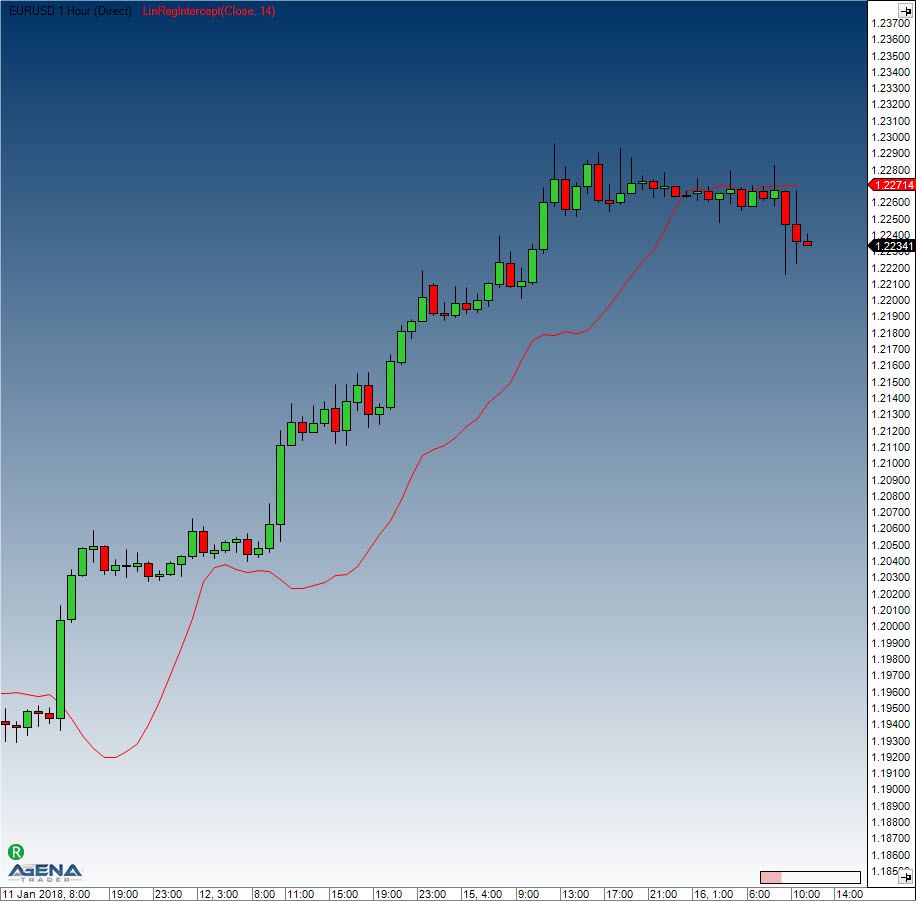# LinRegIntercept

#### Description

The Linear Regression Intercept outputs the value of the regression constant, i.e. the mathematical intersection of where the regression line meets the price axis.
A straight line can be accurately determined with the help of the slope and intercept values.

#### Interpretation

The indicator is not used by itself, but is simply a component of trading systems that analyze trade trends with the help of the linear regression.

#### Usage

LinRegIntercept(int period)
LinRegIntercept(IDataSeries inSeries, int period)
LinRegIntercept(int period)[int barsAgo]
LinRegIntercept(IDataSeries inSeries, int period)[int barsAgo]

#### Return value

double
When using this method with an index (e.g. LinRegIntercept(14)[int barsAgo] ), the value of the indicator will be issued for the referenced bar.

#### Parameters

inSeries Input data series for the indicator
period Number of bars included in the calculations

#### VisualizationLinRegIntercept

#### Example

//Show current value
Print("The current value of the regression constant is: " + LinRegIntercept(14));